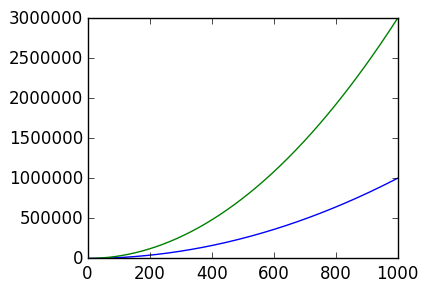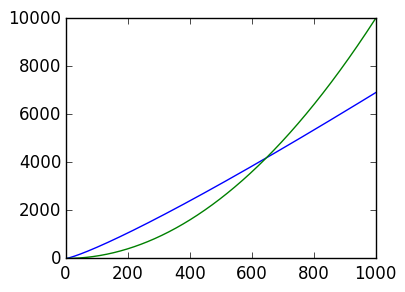# Computational cost and complexity¶

Having created some algorithms (Given's rotations for QR, Gaussian Elimination with Pivoting for PLU), we now turn to studying the properties of these algorithms, so that we can use them in a reliable manner. The possible properties to study include

Scientific Computing Complexity Theory Numerical Analysis
Timings Operation count Stability
Storage cost Complexity Convergence
Error tests

## Timing¶

The simplest test is to just time the algorithm. In Julia, this is possible using thr @time macro:

In :
n=1000
A=rand(n,n)

# Timing

@time lu(A);
@time qr(A);

  0.047212 seconds (38 allocations: 22.897 MB)
0.192263 seconds (38 allocations: 31.068 MB, 19.55% gc time)


We can infer from this calculation that QR takes roughly 3.5x as long as PLU. Doubling the value n verifies this observation:

In :
n=2000
A=rand(n,n)

# Timing

@time lu(A);
@time qr(A);

  0.241879 seconds (38 allocations: 91.569 MB, 2.87% gc time)
0.898618 seconds (38 allocations: 123.170 MB, 3.44% gc time)


## Errors¶

We can test the algorithms error. For example, we have:

In :
n=1000
A=rand(n,n)
Q,R=qr(A)
maximum(abs(Q*R-A))

Out:
1.7208456881689926e-14
In :
L,U,p=lu(A)
P=eye(n)[:,p]
maximum(abs(P*L*U-A))

Out:
2.0650148258027912e-14

We observe that QR is slightly more accurate than PLU for random matrices.

## Operation count¶

It is too prohibitive to count total number of operations, and indeed different operations have different costs. So we use a short hand and count only the Floating Point Operations (FLOPs): this is the number of floating point operations such as addition, division, etc. (Note: Other conventions count an addition combined with a multiplication as a single FLOP, but we won't use that.)

The following is the number of FLOPs per line for backsubstitution. The only floating point operations are on lines 9 and 12:

In :
# back substitution for upper triangular matrices U\b
function backsubstitution(U,b)
n=size(U,1)

x=zeros(n)  # n^2 operations but 0 FLOPS
for k=n:-1:1      # n times
r=b[k]
for j=k+1:n   # n-k times
r = r- U[k,j]*x[j] # one multiplication + one addition
# 2 FLOPS
end
x[k]=r/U[k,k]          # 1 division
# 1 FLOP
end
x
end

Out:
backsubstitution (generic function with 1 method)

However, the for loops mean these lines are called multiple times. Therefore, to determine the true complexity we have to count the number of operations for each for loop. the outer for look is called n times, and the inner for loop for j running from k+1 to n. Thus we get:

Total number of FLOPS for backsubstitution: $$\sum_{k=1}^n \left(\hbox{(# of FLOPs on line 12)} + \sum_{j=k+1}^n \hbox{(# of FLOPs on line 9)} \right)$$ $$= \sum_{k=1}^n \left(1 + \sum_{j=k+1}^n 2 \right)= \sum_{k=1}^n \left(1 + 2 (n-k) \right)= n + 2n^2 - 2\sum_{k=1}^n k = n + 2n^2 - 2 {n^2-n \over 2}$$ $$= n^2+2n$$

## Big-O and little-o notation¶

We now introduce big-o and little-o notation, which simplify expressing algorithm costs. We write (Big-O)

$$f(n) = O(\alpha(n))$$

as $n \rightarrow \infty$ if and only if there exists a constant $C > 0$ and $N > 0$ such that for all $n\geq N$

$$|{f(n)}| \leq C |\alpha(n)|.$$

For example, $n^2 + 2n = O(n^2)$ since $n^2 + 2n \leq 3 n^2$. We can see this graphically:

In :
using PyPlot

n=linspace(1.,1000.,1000)
plot(n,n.^2 + 2n)
plot(n,3n.^2);We write (little-O)

$$f(n) = o(\alpha(n))$$

as $n \rightarrow \infty$ if and only if for every constant $C > 0$ there exists an $N > 0$ such that for all $n\geq N$

$$|{f(n)}| < C |\alpha(n)|.$$

Thus we have $n^2 + 2n = o(n^3)$. Or another example is $n \log n = o(n^2)$. Here we see this pictorially: for small $C = 0.01$, $0.01 n^2$ still exceeds $n \log n$ when $n$ is sufficiently large:

In :
n=linspace(1.,1000.,1000)
plot(n,n.*log(n))
plot(n,0.01n.^2)Out:
1-element Array{Any,1}:
PyObject <matplotlib.lines.Line2D object at 0x30f7cd910>